true## Tutor

Dhanori, Pune, India - 411015.Verified2 Students

Referral Discount: Get ₹ 500 off when you make a payment to start classes. Get started by Booking a Demo.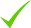IdentityEducation

Know how UrbanPro verifies Tutor details

Identity is verified based on matching the details uploaded by the Tutor with government databases.

## Overview

I am an experienced, qualified tutor with over 5 years of experience in teaching physics and maths.passionate about teaching physics concepts and maths numericals. I have good experience of competitive examinations like NDA, CDSE.

## Languages Spoken

Marathi Mother Tongue (Native)

English Proficient

Hindi Proficient

## Education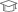fergusson 2013

Bachelor of Science (B.Sc.)pune university 2015

Master of Science (M.Sc.)

Dhanori, Pune, India - 411015

## Verified Info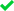ID VerifiedEducation VerifiedPhone VerifiedEmail Verified

Report this Profile

Is this listing inaccurate or duplicate? Any other problem?

Type the letters as shown below *

Please enter the letters as show below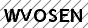## Teaches

Class 10 Tuition1 Student

Class LocationOnline (video chat via skype, google hangout etc)Student's HomeTutor's Home

Years of Experience in Class 10 Tuition

4

NIOS Subjects taught

Mathematics, Science

Board

ICSE, CBSE, State, IGCSE, NIOS, International Baccalaureate

State boards taught

Maharashtra State Board

IB Subjects taught

Physics, Mathematics

CBSE Subjects taught

Science, Mathematics, Social science

ICSE Subjects taught

Physics

IGCSE Subjects taught

Physics

Taught in School or College

Yes

State Syllabus Subjects taught

Science, Mathematics

Class 12 Tuition

Class Location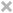Online (video chat via skype, google hangout etc)Student's HomeTutor's Home

Years of Experience in Class 12 Tuition

5

NIOS Subjects taught

Physics, Geography

Board

State, NIOS, ISC/ICSE, CBSE

ISC/ICSE Subjects taught

Physics

CBSE Subjects taught

Physics

Taught in School or College

No

State Syllabus Subjects taught

Mathematics, Physics

Class 9 Tuition

Class LocationOnline (video chat via skype, google hangout etc)Student's HomeTutor's Home

Years of Experience in Class 9 Tuition

5

Board

ICSE, CBSE, State, NIOS

CBSE Subjects taught

Science, Mathematics

ICSE Subjects taught

Physics

Taught in School or College

Yes

State Syllabus Subjects taught

Science, Mathematics

Class 11 Tuition

Class LocationOnline (video chat via skype, google hangout etc)Student's HomeTutor's Home

Years of Experience in Class 11 Tuition

5

NIOS Subjects taught

Mathematics, Physics, Geography

Board

State, NIOS, ISC/ICSE, CBSE

ISC/ICSE Subjects taught

Physics, Mathematics

CBSE Subjects taught

Physics, Mathematics

Taught in School or College

No

State Syllabus Subjects taught

Mathematics, History, Physics, Geography

Class 8 Tuition1 Student

Class LocationOnline (video chat via skype, google hangout etc)Student's HomeTutor's Home

Years of Experience in Class 8 Tuition

5

Board

ICSE, State, CBSE

CBSE Subjects taught

Science, Mathematics

ICSE Subjects taught

Mathematics, Physics, Chemistry

Experience in School or College

I have done my internship from reputed school and it was good experience. I am associated with coaching institute where I am teaching Physics for 11th and 12th std from last 4 years.

Taught in School or College

Yes

State Syllabus Subjects taught

Science, Mathematics

Class 7 Tuition

Class LocationOnline (video chat via skype, google hangout etc)Student's HomeTutor's Home

Years of Experience in Class 7 Tuition

5

Board

ICSE, State, CBSE

State boards taught

Maharashtra State Board

CBSE Subjects taught

Mathematics

ICSE Subjects taught

Mathematics, Physics

Taught in School or College

No

State Syllabus Subjects taught

Science, Mathematics

UPSC Exams Coaching classes

Class LocationOnline (video chat via skype, google hangout etc)Student's HomeTutor's Home

Years of Experience in UPSC Exams Coaching classes

4

Gives

CDS (Combined Defence Service) Exam Coaching, NDA (National Defence Acadamy and Naval Acadamy) Exam Coaching

## Reviews

No Reviews yet! Be the first one to Review

## FAQs

1. Which school boards of Class 10 do you teach for?

ICSE, CBSE, State and others

2. Do you have any prior teaching experience?

Yes

3. Which classes do you teach?

I teach Class 10 Tuition, Class 11 Tuition, Class 12 Tuition, Class 7 Tuition, Class 8 Tuition, Class 9 Tuition and UPSC Exams Coaching Classes.

4. Do you provide a demo class?

Yes, I provide a paid demo class.

5. How many years of experience do you have?

I have been teaching for 4 years.

You can guess the square root with simple logic. For example 81. - sq root is 9 361 is sq root of number whose unit = 1 or 9 Square of 20 is 400 .so u can it's square root of either 11 or 19. If you compare sq of 20 u can easily guess it sq root of 19. If last digit is 4 then it's square root of... ...more

You can guess the square root with simple logic.

For example

81.   - sq root is 9

361 is sq root of number whose unit = 1 or 9

Square of 20 is 400 .so u can it's square root of either 11 or 19.

If you compare sq of 20 u can easily guess it sq root of 19.

If last digit is 4 then it's square root of either 2 or 8

If last digit is 9 then it's square root of either 7 or 3

If last digit is 6 then it's square root of either 4 or 6

And if it's 5 then 5

Dislike Bookmark

Answered on 18/08/2019 Learn CBSE/Class 9/Mathematics/Surface Area and Volumes/NCERT Solutions/Exercise 13.7

given h = 15 cm volume of cone = 1570 to find- radius (r) formula- volume of cone = 1570 = 3.14 * * 15 /3 1570 *3 = 3.14 * 15 * = 1570 * 3 / 3.14 * 15 =100 taking square roots on both sides , we get r = 10 cm ...more

given

h = 15 cm

volume of cone = 1570

formula-

volume of cone =

1570     = 3.14 *  * 15 /3

1570 *3 =  3.14 * 15 *

=   1570 * 3 / 3.14 * 15

=100

taking square roots on both sides , we get

r = 10 cm

Dislike Bookmark

Answered on 18/08/2019 Learn CBSE/Class 9/Mathematics/Surface Area and Volumes/NCERT Solutions/Exercise 13.7

Diameter of the conical pit = 3.5m radius= 1.75m height=12m volume of cone= π h /3 = 3.14 * 1.75^2 * 12 /3 =115.39/3 = 38.46 volume of cone = 38.46 m^3 1= 1000 L = 1 kilolitre hence , 38.5 = 38.5 kilolitrs ...more

Diameter of the conical pit = 3.5m

height=12m

volume of cone= π h /3

= 3.14 * 1.75^2 * 12  /3

=115.39/3

= 38.46

volume of cone = 38.46 m^3

1= 1000 L = 1 kilolitre

hence ,

38.5  = 38.5 kilolitrs

Dislike Bookmark

Answered on 17/08/2019 Learn CBSE/Class 9/Mathematics/Surface Area and Volumes/NCERT Solutions/Exercise 13.7

1. L^2 = h^2 + r^2 L = slant height h= height r= radius 25^2 = h^2 + 7^2 h^2 = 625 - 49 = 576 after taking square root h = 24 volume of cone = L^2 = h^2 + r^2 = 1/3 * 3.14 *7^2 *... ...more

1.    L^2 = h^2 + r^2              L = slant height   h= height  r= radius

25^2 = h^2 + 7^2

h^2 = 625 - 49 = 576

after taking square root

h = 24

volume of cone =                               L^2 = h^2 + r^2

= 1/3 * 3.14 *7^2 * 24

=3692.64/3

volume of cone  =1230 cm^3

1L = 1000cm^3

Dislike Bookmark

## Teaches

Class 10 Tuition1 Student

Class LocationOnline (video chat via skype, google hangout etc)Student's HomeTutor's Home

Years of Experience in Class 10 Tuition

4

NIOS Subjects taught

Mathematics, Science

Board

ICSE, CBSE, State, IGCSE, NIOS, International Baccalaureate

State boards taught

Maharashtra State Board

IB Subjects taught

Physics, Mathematics

CBSE Subjects taught

Science, Mathematics, Social science

ICSE Subjects taught

Physics

IGCSE Subjects taught

Physics

Taught in School or College

Yes

State Syllabus Subjects taught

Science, Mathematics

Class 12 Tuition

Class LocationOnline (video chat via skype, google hangout etc)Student's HomeTutor's Home

Years of Experience in Class 12 Tuition

5

NIOS Subjects taught

Physics, Geography

Board

State, NIOS, ISC/ICSE, CBSE

ISC/ICSE Subjects taught

Physics

CBSE Subjects taught

Physics

Taught in School or College

No

State Syllabus Subjects taught

Mathematics, Physics

Class 9 Tuition

Class LocationOnline (video chat via skype, google hangout etc)Student's HomeTutor's Home

Years of Experience in Class 9 Tuition

5

Board

ICSE, CBSE, State, NIOS

CBSE Subjects taught

Science, Mathematics

ICSE Subjects taught

Physics

Taught in School or College

Yes

State Syllabus Subjects taught

Science, Mathematics

Class 11 Tuition

Class LocationOnline (video chat via skype, google hangout etc)Student's HomeTutor's Home

Years of Experience in Class 11 Tuition

5

NIOS Subjects taught

Mathematics, Physics, Geography

Board

State, NIOS, ISC/ICSE, CBSE

ISC/ICSE Subjects taught

Physics, Mathematics

CBSE Subjects taught

Physics, Mathematics

Taught in School or College

No

State Syllabus Subjects taught

Mathematics, History, Physics, Geography

Class 8 Tuition1 Student

Class LocationOnline (video chat via skype, google hangout etc)Student's HomeTutor's Home

Years of Experience in Class 8 Tuition

5

Board

ICSE, State, CBSE

CBSE Subjects taught

Science, Mathematics

ICSE Subjects taught

Mathematics, Physics, Chemistry

Experience in School or College

I have done my internship from reputed school and it was good experience. I am associated with coaching institute where I am teaching Physics for 11th and 12th std from last 4 years.

Taught in School or College

Yes

State Syllabus Subjects taught

Science, Mathematics

Class 7 Tuition

Class LocationOnline (video chat via skype, google hangout etc)Student's HomeTutor's Home

Years of Experience in Class 7 Tuition

5

Board

ICSE, State, CBSE

State boards taught

Maharashtra State Board

CBSE Subjects taught

Mathematics

ICSE Subjects taught

Mathematics, Physics

Taught in School or College

No

State Syllabus Subjects taught

Science, Mathematics

UPSC Exams Coaching classes

Class LocationOnline (video chat via skype, google hangout etc)Student's HomeTutor's Home

Years of Experience in UPSC Exams Coaching classes

4

Gives

CDS (Combined Defence Service) Exam Coaching, NDA (National Defence Acadamy and Naval Acadamy) Exam Coaching

No Reviews yet! Be the first one to Review

You can guess the square root with simple logic. For example 81. - sq root is 9 361 is sq root of number whose unit = 1 or 9 Square of 20 is 400 .so u can it's square root of either 11 or 19. If you compare sq of 20 u can easily guess it sq root of 19. If last digit is 4 then it's square root of... ...more

You can guess the square root with simple logic.

For example

81.   - sq root is 9

361 is sq root of number whose unit = 1 or 9

Square of 20 is 400 .so u can it's square root of either 11 or 19.

If you compare sq of 20 u can easily guess it sq root of 19.

If last digit is 4 then it's square root of either 2 or 8

If last digit is 9 then it's square root of either 7 or 3

If last digit is 6 then it's square root of either 4 or 6

And if it's 5 then 5

Dislike Bookmark

Answered on 18/08/2019 Learn CBSE/Class 9/Mathematics/Surface Area and Volumes/NCERT Solutions/Exercise 13.7

given h = 15 cm volume of cone = 1570 to find- radius (r) formula- volume of cone = 1570 = 3.14 * * 15 /3 1570 *3 = 3.14 * 15 * = 1570 * 3 / 3.14 * 15 =100 taking square roots on both sides , we get r = 10 cm ...more

given

h = 15 cm

volume of cone = 1570

formula-

volume of cone =

1570     = 3.14 *  * 15 /3

1570 *3 =  3.14 * 15 *

=   1570 * 3 / 3.14 * 15

=100

taking square roots on both sides , we get

r = 10 cm

Dislike Bookmark

Answered on 18/08/2019 Learn CBSE/Class 9/Mathematics/Surface Area and Volumes/NCERT Solutions/Exercise 13.7

Diameter of the conical pit = 3.5m radius= 1.75m height=12m volume of cone= π h /3 = 3.14 * 1.75^2 * 12 /3 =115.39/3 = 38.46 volume of cone = 38.46 m^3 1= 1000 L = 1 kilolitre hence , 38.5 = 38.5 kilolitrs ...more

Diameter of the conical pit = 3.5m

height=12m

volume of cone= π h /3

= 3.14 * 1.75^2 * 12  /3

=115.39/3

= 38.46

volume of cone = 38.46 m^3

1= 1000 L = 1 kilolitre

hence ,

38.5  = 38.5 kilolitrs

Dislike Bookmark

Answered on 17/08/2019 Learn CBSE/Class 9/Mathematics/Surface Area and Volumes/NCERT Solutions/Exercise 13.7

1. L^2 = h^2 + r^2 L = slant height h= height r= radius 25^2 = h^2 + 7^2 h^2 = 625 - 49 = 576 after taking square root h = 24 volume of cone = L^2 = h^2 + r^2 = 1/3 * 3.14 *7^2 *... ...more

1.    L^2 = h^2 + r^2              L = slant height   h= height  r= radius

25^2 = h^2 + 7^2

h^2 = 625 - 49 = 576

after taking square root

h = 24

volume of cone =                               L^2 = h^2 + r^2

= 1/3 * 3.14 *7^2 * 24

=3692.64/3

volume of cone  =1230 cm^3

1L = 1000cm^3

Dislike Bookmark

Ganesh Wadkar describes himself as Tutor. He conducts classes in Class 10 Tuition, Class 11 Tuition and Class 12 Tuition. Ganesh is located in Dhanori, Pune. Ganesh takes at students Home and Regular Classes- at his Home. He has 5 years of teaching experience . Ganesh has completed Bachelor of Science (B.Sc.) from fergusson in 2013 and Master of Science (M.Sc.) from pune university in 2015. He is well versed in English, Hindi and Marathi.

•• Want to learn from Ganesh Wadkar?

• Contact Now
X

Recommended Profiles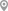Dhanori, PuneJyotikuchi, GuwahatiDhanori, PuneBaner, PuneNanded, PuneDhanori, Pune

1500/1500Certified

The Certified badge indicates that the Tutor has received good amount of positive feedback from Students.

Different batches available for this Course